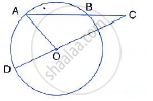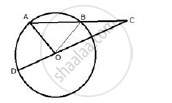Share

# In the Figure, Ab is the Chord of a Circle with Centre O and Doc is a Line Segment Such that Bc = Do. If ∠C = 20°, Find Angle Aod. - Mathematics

Course

#### Question

In the figure, AB is the chord of a circle with centre O and DOC is a line segment such that BC = DO. If ∠C = 20°, find angle AOD.#### SolutionJoin OB,
In ΔOBC,
BC = OD = OB (Radii of the same circle)
∴ ∠BOC = ∠BCO = 20°
And Ext. ∠ABO =  ∠BCO + ∠BOC
⇒ Ext..∠ABO = 20° + 20°  = 40° …… (i)
In ΔOAB,
OA = OB (radii of the same circle)
∴ ∠OAB = ∠OBA = 40° (from (i)
∠AOB = 180°  -  ∠OAB - ∠OBA
⇒ ∠AOB  = 180°  - 40° - 40° = 100°
Since DOC is a straight line
∴ ∠AOD+ ∠AOB + ∠BOC  = 180°
⇒ ∠AOD + 100° + 20° = 180°
⇒ ∠AOD = 180° - 120°
⇒ ∠AOD = 60°

Is there an error in this question or solution?